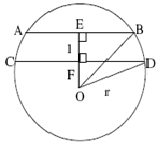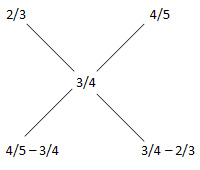# Sample Aptitude Questions of Huawei

1. A farmer has decided to build a wire fence along one straight side of his property. For this, he planned to place several fence-posts at 6 m intervals, with posts fixed at both ends of the side. After he bought the posts and wire, he found that the number of posts he had bought was 5 less than required. However, he discovered that the number of posts he had bought would be just sufficient if he spaced them 8 m apart. What is the length of the side of his property and how many posts did he buy?
1. 100 m, 15
2. 100 m, 16
3. 120 m, 15
4. 120 m, 16
Since it is given that length is sufficient for 6mt. and 8 mtr. gaps so it must be a common multiple of 6 & 8 which out of choices can be 120 meters only. Now as a concept to generate 15 gaps (= 120/8), you need 16 poles. So, answer is 4th option.
2. The number of ways in which a committee of 3 ladies and 4 gentlemen can be appointed from a meeting consisting of 8 ladies and 7 gentlemen, if Mrs. X refuses to serve in a committee if Mr. Y is its member, is
1. 1960
2. 3240
3. 1540
4. None of these
Required no. of ways = No. of ways of selecting 3 ladies & 4 gentlemen - No. of ways of selecting them when X & Y got selected
= 8C3 × 7C4 - 7C2 × 6C3 = 1960 - 420 = 1540.
Thus, option C is correct.
1. Ramesh takes twice as much time as Mahesh and thrice as much time as Suresh to complete a job. If working together, they can complete the job in 4 days, then the time taken by each of them separately to complete the work is
1. 36, 24 and 16 day
2. 20, 16 and 12 days
3. 24, 12 and 8 days
4. None of these
We are given that R = 2M = 3S. This means that Suresh is thrice efficient than Ramesh and Mahesh is twice efficient than Ramesh.
So let us assume that Ramesh can do 1 unit work per day. so Mahesh will do 2 units per day and Suresh will do 3 units of the work per day.
So the total work done by all three in one day is 6 units. Now they together take 4 days to complete the work.
So the total work is 6 x 4 = 24 units and individually Ramesh, Mahesh and Suresh will take 24 days, 12 days and 8 days respectively.
2. Three sides of a triangular field are 20 meters, 21 meters and 29 meters long, respectively. The area of the field is
1. 215 sq m
2. 230 sq m
3. 210 sq m
4. None of these
The given sides are 20,21,and 29 and 202+212=292
Since it is a right angled triangle, so area = 1/2 * 20 * 21 = 210 sq m.
3. Find the greatest number that will divide 964, 1238 and 1400 leaving remainders 41, 31 and 51, respectively.
1. 71
2. 81
3. 61
4. 73
We have 964 – 41 = 923, 1238 – 31 = 1207 and 1400 – 51 = 1349.
So the required number is the HCF of 923, 1207 and 1349 which is 71. Hence 1st option.
4. In a rhombus ABCD the diagonals AC and BD intersect at the point (3, 4). If the point 'A' is (1, 2), the diagonal BD has the equation
1. x – y – l = 0
2. x – y + l = 0
3. x + y – l = 0
4. x + y – 7 = 0
Slope of AC = (2-4)/(1-3) = 1. As diagonals in a rhombus bisect each other at 90°, so slope of BD = -1.
Also diagonal BD has to lie on the point (3, 4), so equation of the line is y - 4 = - 1(x - 3) ⇒ x + y – 7 = 0.
5. A circle has two parallel chords of lengths 6 cm and 8 cm. If the chords are 1 cm apart and the centre is on the same side of the chords, then the diameter of the circle is of length:
1. 5 cm
2. 6 cm
3. 10 cm
4. 12 cmIn the given figure, EB = 3 cm, FD = 4 cm, EF = 1 cm
Let OF = a cm
In triangle OFD, r2 =a2+42 in triangle OEB, r2 =(a+1)2+32
Solving the above two equations, we get a = 3cm
Putting the value of a in any of the above equation, we get r = 5 cm
Therefore, diameter = 10 cm.
6. An express train travelled at an average speed of 100 kmph, stopping for 3 minutes after every 75 km. A local train travelled at a speed of 50 kmph, stopping for 1 minute after every 25 km. If the trains began travelling at the same time, how many kilometres did the local train travel in the time it took the express train to travel 600 km?
1. 900 km
2. 307.5 km
3. 1200 km
4. 100 km
For 600 kms. The Express train needs 6 hrs. for the distance travelled @ 100 kmph. and it will stop for ( 600/75)=8.
So there must be 7 stoppages in between where it stops for 3 min. each totalling upto 21 min. So total time will be 6 hrs.21 min.
Now the Local train travelling @ 50 kmph. will cover 300 kms. in 6 hrs. and in rest 9 min. it will travel 7.5 kms.( 300/25=12 stoppages where it will stop for 12 min. So, 21- 12 = 9 ).
The total distance becomes 300 + 7.5 = 307.5 km.
ALTERNATE SOLUTION We can also think that since speed of express train is double the local speed, so local train will tavel less than 600 km, Only option b has such option and hence is the required answer
7. Two alloys contain zinc and copper in the ratio of 2 : 1 and 4 : 1. In what ratio the two alloys should be added together to get as new alloy having zinc and copper in the ratio of 3 : 1?
1. 7:5
2. 5:7
3. 3:5
4. None of the above